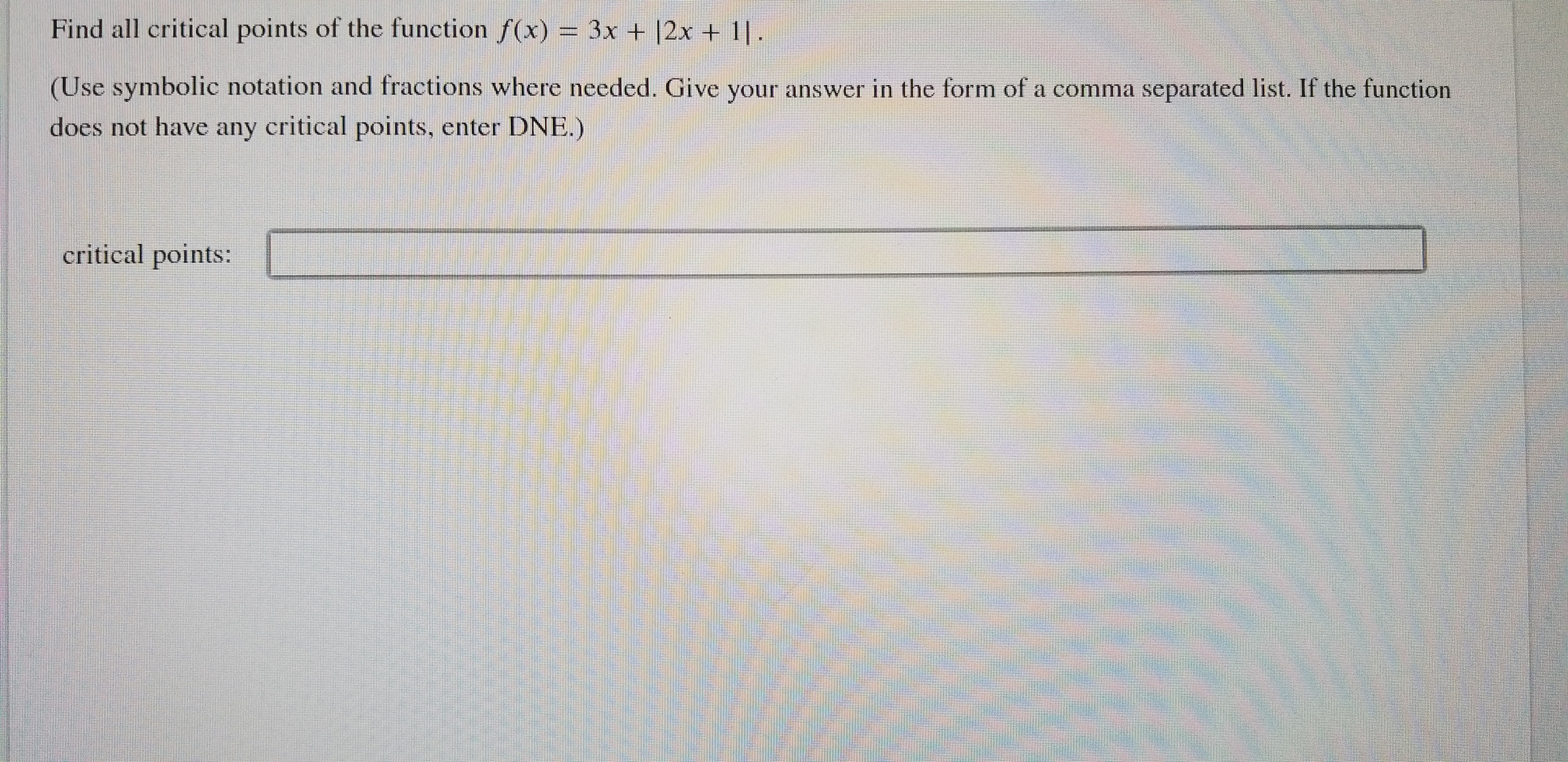# Find all critical points of the function f(x)3x + 12x + 11.(Use symbolic notation and fractions where needed. Give your answer in the form of a comma separated list. If the functiondoes not have any critical points, enter DNE.)critical points:

Question
17 viewshelp_outlineImage TranscriptioncloseFind all critical points of the function f(x) 3x + 12x + 11. (Use symbolic notation and fractions where needed. Give your answer in the form of a comma separated list. If the function does not have any critical points, enter DNE.) critical points: fullscreen
check_circle

Step 1

|2x + 1| = 2x + 1 if 2x + 1 ≥  0 i.e. x ≥  -1/2

|2x + 1| = -(2x + 1) if 2x + 1 < 0 i.e. x > -1/2

Step 2

Hence, f(x) = 3x + |2x + 1| = 3x + 2x + 1 = 5x + 1 if x ≥ - 1/2 and

f(x) = 3x - (2x + 1) = x - 1 if x < -1/2

Step 3

Critical points are minima, maxima, and points where f'(x)=0.

Since both the branches of f(x) are polynomial function in x,  there is no point where f'(x) = 0.

...

### Want to see the full answer?

See Solution

#### Want to see this answer and more?

Solutions are written by subject experts who are available 24/7. Questions are typically answered within 1 hour.*

See Solution
*Response times may vary by subject and question.
Tagged in

### Calculus